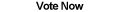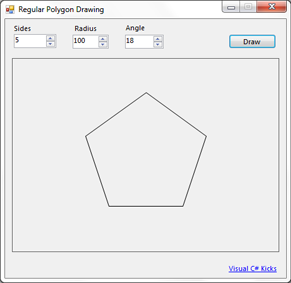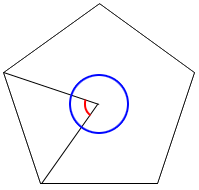Home Articles Components Code Snippets Links# C# Drawing Regular Polygons## Regular Polygons

Drawing polygons in C# is relatively easy thanks to GDI+. As far as drawing regular polygons (polygons with edges of the same size) it gets slightly tricky because we need to calculate the polygon vertices.

## Math

The math for a regular polygon is not too difficult. Since the each of the sides are the same length, this means the angle from the center of the polygon to each vertex in an edge is the same for every edge.Thus we can calculate the interior angle of each edge by diving the total number of degrees in a circle (360) by the number of sides. So for our example above, a pentagon (5-sided polygon) would have interior angles of 360/5 = 72 degrees.

How do we calculate the vertices from the interior angle? Easy, we just need our degree to coordinate code snippet. The function takes in as arguments: an origin (the center of the polygon), a radius, and a degree. The function simply uses trigonometry to figure out how long the hypotenuse of a right triangle would be starting at the origin at the given angle. You can look at the code in the link above or in the sample project at the bottom of the page.

It is now super easy to calculate the vertices of the polygon. With a simple loop, we can use the degree to coordinate function at each angle interval. The code ends up looking like this:

```private Point[] CalculateVertices(int sides, int radius, int startingAngle, Point center)
{
if (sides < 3)
throw new ArgumentException("Polygon must have 3 sides or more.");

List<Point> points = new List<Point>();
float step = 360.0f / sides;

float angle = startingAngle; //starting angle
for (double i = startingAngle; i < startingAngle + 360.0; i += step) //go in a full circle
{
angle += step;
}

return points.ToArray();
}```

## Drawing

Drawing the polygon on an image is as simple as using the DrawPolygon function in the System.Drawing.Graphics class:

```Bitmap polygon = new Bitmap(canvasSize.Width, canvasSize.Height);
using (Graphics g = Graphics.FromImage(polygon))
{
g.SmoothingMode = System.Drawing.Drawing2D.SmoothingMode.HighQuality;
g.DrawPolygon(Pens.Black, verticies);
}```

The .NET Framework will take care of the actual drawing logic

## Final Notes

Few things to note. First of all, the function returns Point objects, depending on how accurate you need the polygon-drawing to be you could use PointF instead. Both DegreesToXY and DrawPolygon already support PointF.

For the math, the angles are all in degrees and go counter clockwise starting with zero at the right-most midpoint of the circle.

Finally below is a sample C# program that wraps a nice user interface so you can view the regular polygon drawing code in action.

Back to C# Article List